Electron. J. Diff. Equ., Vol. 2016 (2016), No. 88, pp. 1-15.

### Infinitely many solutions for fractional Schrodinger equations in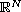Caisheng Chen

Abstract:
Using variational methods we prove the existence of infinitely many solutions to the fractional Schrodinger equation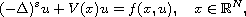where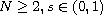.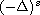stands for the fractional Laplacian. The potential function satisfies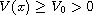. The nonlinearity f(x,u) is superlinear, has subcritical growth in u, and may or may not satisfy the (AR) condition.

Submitted January 31, 2016. Published March 30, 2016.
Math Subject Classifications: 35R11, 35A15, 35J60, 47G20.
Key Words: Fractional Schrodinger equation; variational methods; (PS) condition; (C)c condition

Show me the PDF file (295 KB), TEX file, and other files for this article.Caisheng Chen College of Science, Hohai University Nanjing 210098, China email: cshengchen@hhu.edu.cn